### Home > CALC > Chapter 1 > Lesson 1.2.5 > Problem1-83

1-83.
1. If f(x) = x2 + 5x and g(x) = x + 3, evaluate each of these expressions. Homework Help ✎

1. f(−2)

2. g(−2)

3. f(g(−2))

4. g(f(−2))

5. f(f(−2))

6. g(g(−2))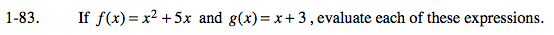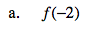f(−2) = (−2)2 + 5(−2) =

-16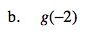g(−2) = (−2) + 3 =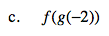f(g(−2)) = f(part (b)).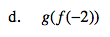g(f(−2)) = g(part (a)).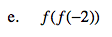Refer to hint (d).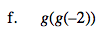Refer to hint (c).